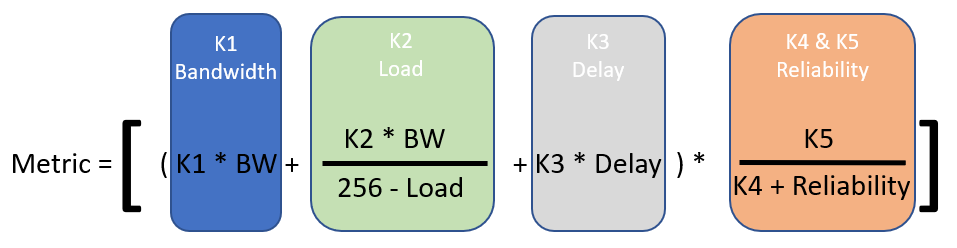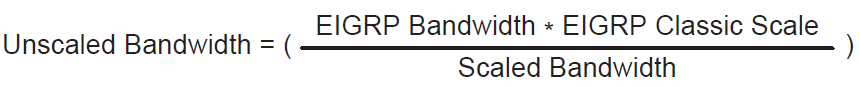# EIGRP Metric Calculation and Formulas

Enhanced Interior Gateway Routing Protocol (EIGRP) is a Cisco proprietary routing that they developed to address the limitations of other distance vector routing protocols such as RIP by being able to implement unequal-cost load balancing. EIGRP metric can support networks even if they are 255 hops away and has quick convergence features.

One feature of EIGRP is that it uses various factors to calculate the metric for its path. EIGRP metric values, by default, utilize bandwidth value and delay value but also consider interface load and reliability. Below is the formula for EIGRP classic metric calculation:## What are EIGRP K-Values?

The metric calculation in EIGRP considers the K-values used to calculate the best route. The value can be between 0-255 and independently set, with reference to what is considered for the cost calculation for each route. If an attribute is not considered, the K-value for that should be equal to zero, but if it is considered, the K-value should be equal to one.

As stated, the maximum K-value for EIGRP metrics is 255, so the ability to manipulate what route metric is defined during the path calculation. For example, if you want the Bandwidth to be a priority over Delay, you can configure the K-value of K1 to be higher than K3.

EIGRP offers flexibility in deciding which route to prioritize by setting the K-values of the preferred attribute in the routing domain. It is important to remember that EIGRP neighbors must have matching K-values.

## EIGRP Classic Metric Formula with Default K-values

When considering the default K-values in calculating the EIGRP metric calculation formula of the route, K1 and K3 should always be 1, and K2, K4, and K5 are zero. The EIGRP formula is derived from the IGRP metric formula, then multiplied by 256 to change the calculation from 24 to 32 bits. Bandwidth is the slowest link referenced to a 10 Gbps link (107). The link speed is from the configured Bandwidth on the interface, and Delay values are the quantity of the total measure of path delay in microseconds.By calculating the above equation considering all the default K-values, we will come up with the EIGRP Classic Metric formula.The table below shows the default EIGRP Interface Metrics that we can use for the Classic Metric formula:

 Interface Type Link Speed (Kbps) Delay Metric Serial 64 20,000 μs 40,512,000 T1 1544 20,000 μs 2,170,031 Ethernet 10,000 1000 μs 281,600 FastEthernet 100,000 100 μs 28,160 GigabitEthernet 1,000,000 10 μs 2816 10 GigabitEthernet 10,000,000 10 μs 512

Using the Classic EIGRP Metric formula, let us compute the EIGRP metric of the network below passing through R1> R3>R4 to the destination network.The link speed is 1 Gigabit, so it is 1,000,000 Kbps for the minimum Bandwidth and the total Delay is 30μs. Therefore, the metric would be 3328.The route metrics can be checked using the ‘show ip eigrp topology’ command. The network and subnet mask are added at the end of the command to view the detailed EIGRP information of the route. The show command below shows the details of the EIGRP route from R1 to the 10.2.2.0/24 network. You can see that the metric calculated, 3328, is the same as the metric in the output below:

```R1#sh ip eigrp topology 10.2.2.0/24
EIGRP-IPv4 Topology Entry for AS(1)/ID(10.0.0.2) for 10.2.2.0/24
State is Passive, Query origin flag is 1, 1 Successor(s), FD is 3328
Descriptor Blocks:
10.0.0.1 (GigabitEthernet1), from 10.0.0.1, Send flag is 0x0
Composite metric is (3328/3072), route is Internal
Vector metric:
Minimum bandwidth is 1000000 Kbit
Total delay is 30 microseconds
Reliability is 255/255
Minimum MTU is 1500
Hop count is 2
Originating router is 10.2.2.2```

## Wide Metrics

EIGRP specifications originally measured Delay to be 10 microseconds and the Bandwidth in kbps which is not scalable with interfaces with higher speeds. With this issue, EIGRP wide metrics formula has a second set that addresses the scalability limitations with higher-speed interfaces by adding a K-value in the EIGRP metric calculations, which is K6 that measures the jitter, energy, and other attributes in the future.

##EIGRP wide metrics scale by 65,536 to support 655 Tbps (65,536 × 107) interface speed without any scalability issue. The scaled EIGRP wide metrics formula takes scalability and latency, which is the total delay in picoseconds, into account.

## EIGRP Metric Backward Compatibility

The EIGRP wide metrics were developed with the ability to be backward compatible. As long as K1 through K5 are the same and K6 is not provided or is equal to zero, the classic metric and wide metrics can establish adjacency between routers.

Moreover, EIGRP can detect when the peering between two routers uses classic metric, thus unscaling a metric from the formula.

##Download our Free CCNA Study Guide PDF for complete notes on all the CCNA 200-301 exam topics in one book.

We recommend the Cisco CCNA Gold Bootcamp as your main CCNA training course. It’s the highest rated Cisco course online with an average rating of 4.8 from over 30,000 public reviews and is the gold standard in CCNA training: